### Ecological Archives E082-016-A2

Douglas C. Speirs and William S. C. Gurney. 2001. Population persistence in rivers and estuaries. Ecology 82:1219-1237.

Appendix B. Discrete space-time models.

### Logistic growth in a one-dimensional habitat

To avoid the computationally onerous task of solving Eqs. (1) and (12) numerically, we use an equivalent discrete space-time representation described by Gurney et al. (1998). We divide space into a contiguous series of equally spaced quadrats of width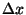and denote the average population density in quadrat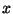at time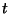by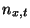. This density distribution is updated at intervals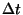according to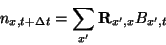(B.1)

where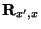is the redistribution matrix and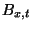represents the number of survivors and descendents of the population of quadratat timewho are present at time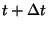. Following Gurney et al. (1998) we equatewith the solution of the logistic equation, so that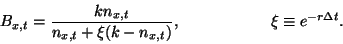(B.2)

Well away from the domain boundaries we again follow Gurney et al. (1998) and write the redistribution matrixas a displaced tent distribution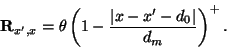(B.3)

The coefficients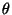,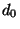, and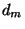are chosen so that the distribution is properly normalized with a mean displacement of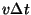and a displacement variance of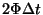. That is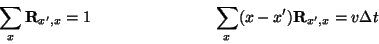(B.4)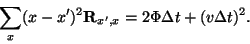(B.5)

At the boundaries we use the method of images to define an appropriately modified redistribution matrix. In the case of the closed boundary we generate a mirror image of the distribution defined by Eqs. (B.3) to (B.5) reflected in a plane midway between the last node in the domain and its (notional) left-hand neighbor. The part of this reflection within the domain is added to the original distribution. The area thus added is exactly equal to the part of the original distribution falling outside the domain, so the distribution remains properly normalized. At the open boundary we employ a related strategy in which that part of the reflection falling within the domain is subtracted from the original distribution, and added to that falling beyond the open boundary.

### Logistic growth in a two-dimensional habitat

This model explicitly represents both distance from the river source () and depth below the river surface (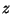), so we denote position by a vector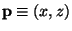. We define a contiguous series of rectangular quadrats of widthand depth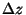and denote the average population density in quadrat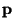at timeby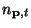. This density distribution is updated at intervalsaccording to a generalisation of Eq. (B.1)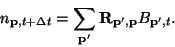(B.6)

The local growth function is given by Eq. (B.2), and the redistribution matrix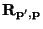is a generalisation of the tent distribution given in Eq. (B.3)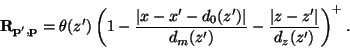(B.7)

For each originating depth (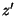) the coefficients,,and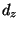are chosen so as to ensure that the distribution is properly normalised and has the appropriate mean and variance for the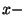and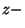displacements, that is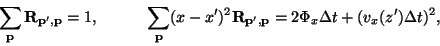(B.8)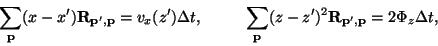(B.9)

where the advection velocity (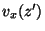) is related to depth and the overall average river velocity by Eq. (14).

### A two-dimensional tidally driven habitat

In a tidally driven situation, the deterministic flow fields vary with time and it seems obvious that the redistribution matrixmust be time-dependent likewise. However, if we set the update increment to exactly one tidal period,represents the residual motion averaged over the tidal cycle. If we further (strategically) ignore the spring-neap cycle,becomes time-independent.

Population processes typically have timescales of days rather than hours, so we expect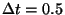day to be a viable choice of update increment. To check this we repeated a selection of our earlier simulations (which used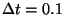day) and observed that the results were weakly affected by the increased. This result is in accordance with the findings of Gurney et al. (1998) in the context of a discrete spatial predator-prey model.

Withset equal to the tidal period, our model is defined by Eqs. (B.6) and (B.7) with the coefficients of the redistribution matrix chosen so that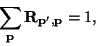(B.10)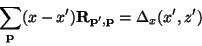(B.11)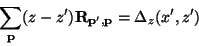(B.12)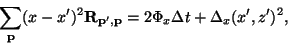(B.13)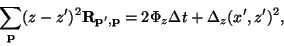(B.14)

In these equations,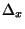and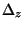represent the x- and z-components of the net displacement of a neutrally buoyant particle starting at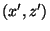over exactly one tidal cycle. We compute this quantity by tracking such a particle in the flow field defined by Eqs. (C.16) and (C.19) using a fourth order Runge-Kutta algorithm (Press et al. 1986) with a time increment of 10 minutes.

[Back to E082-016]